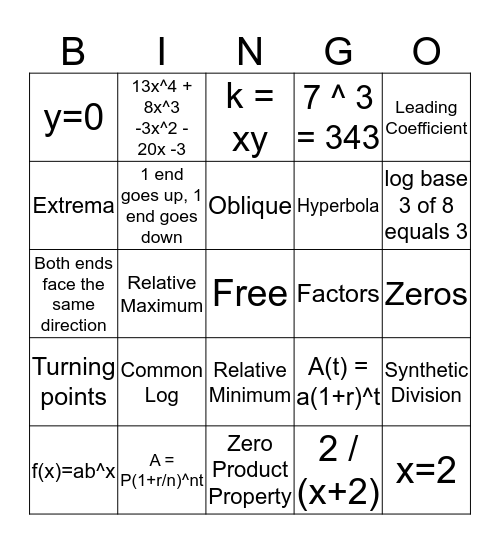# Everything Algebra IIThis bingo card has a free space and 24 words: Common Log, A = P(1+r/n)^nt, Oblique, f(x)=ab^x, Leading Coefficient, y=0, Extrema, 13x^4 + 8x^3 -3x^2 - 20x -3, Factors, Relative Minimum, Hyperbola, Both ends face the same direction, A(t) = a(1+r)^t, Synthetic Division, 1 end goes up, 1 end goes down, 2 / (x+2), Zeros, k = xy, log base 3 of 8 equals 3, Relative Maximum, x=2, Turning points, 7 ^ 3 = 343 and Zero Product Property.

## Play Online